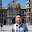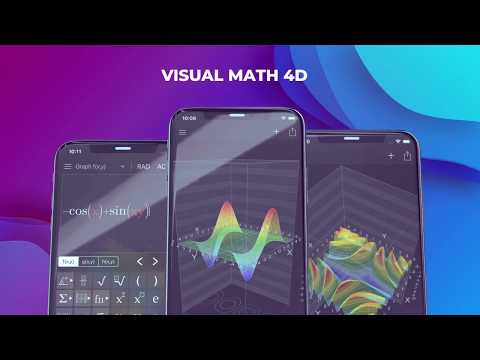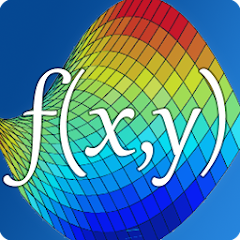# Visual Math 4D

In-app purchases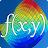4.5
973 reviews
100K+Everyone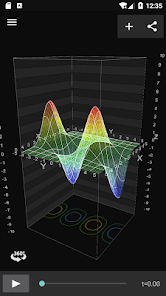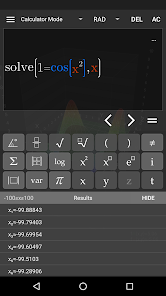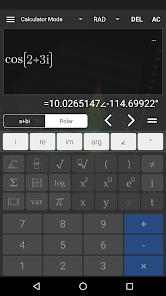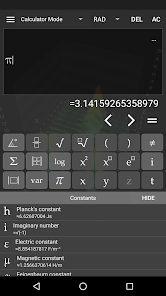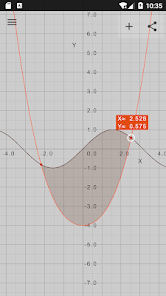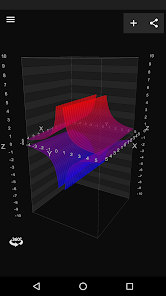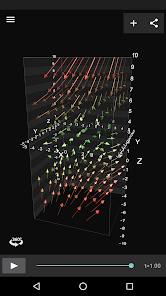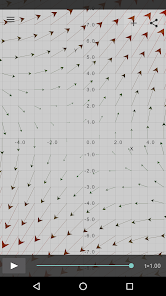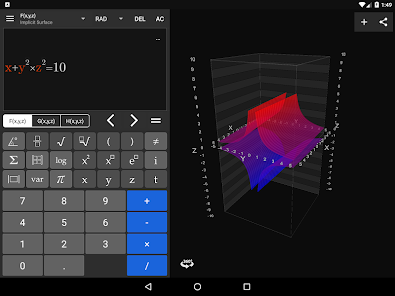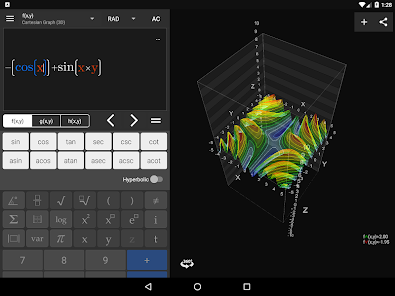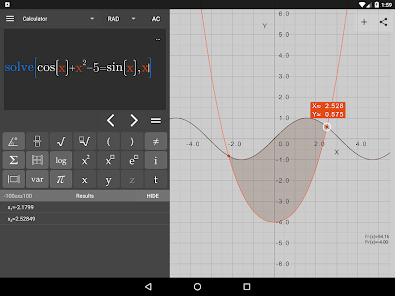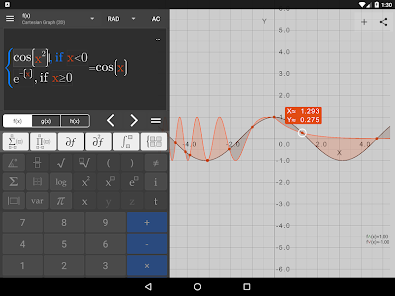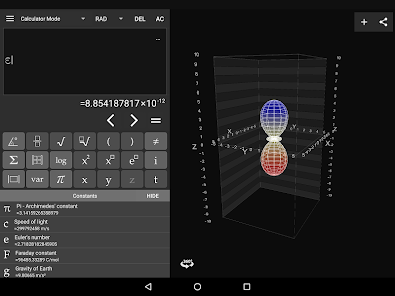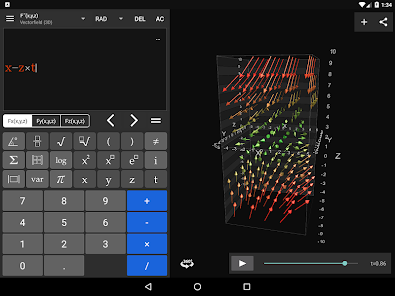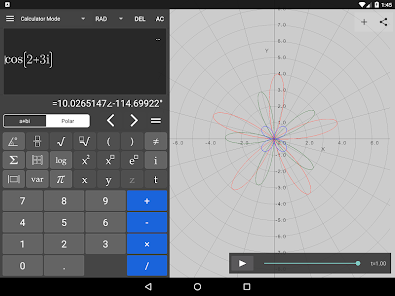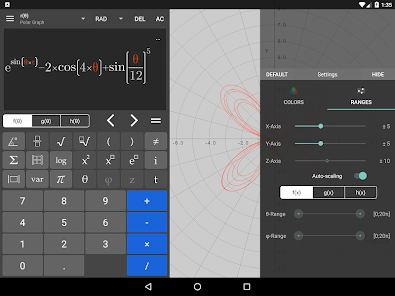Visual Math 4D is a graphical calculator that allows you to visualize and solve your mathematical equations. It supports various types of equations, such as spherical, parametrical, polar and cartesian and implicit equations, which can be visualized and animated in 2D and 3D. You can also plot and animate vector fields in 2D and 3D.

Features:
- solve equations and visualize their intersections
- plot cartesian functions with intersections
- plot polar- and spherical functions
- plot parametrical equations
- plot complex functions (draw real part & imaginary part)
- plot vector fields in 2D and 3D
- plot implicit equations in 2D and 3D
- plot contours of your functions
- complex numbers
- vectors & matrices
- truth- and value tables
- trigonometrical and hyperbolic functions
- piecewise-defined functions
- logarithmic functions
- logical & binary operators
- definite integral
- n-th derivations
- statistical functions
- physical and mathematical constants with units
- animate variables
- share content with other apps
- no network connection required
- and a lot more...

The app is easy to use and helps students and engineers to visualize and solve their mathematical equations.
Updated on
Feb 26, 2019

## Data safetyNo information available
4.5
973 reviews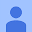August 28, 2019
Despite the good looks, lacks real polish and intuitiveness. Ex, pressing the VAR let brings up a list of variables, as expected, but then pressing the back key exits the entire app. What if I pressed the VAR key by accident? That's like being forced to eat a dish one ordered by mistake - this behavior has no place anywhere in a modern GUI. It's not clear how to access any of the trig functions from the CALC menu either; it should be. This app needs help.
13 people found this review helpful
appnova
September 7, 2019
Thank you for your feedback. We still working on a better back-button handling and a lot more. Kind regards Ronny from appnovaApril 5, 2019
Really fantastic. I use this when I need a quick intuition of higher dimensions or different coordinate systems. This calculator is super fast, has any type of graph you might need for multivariable and vector calculus, applied math+physics, and arbitrary functions in Cartesian/Spherical/Polar spaces. Definitely worth it! High quality, easy to use, beautiful interface.
11 people found this review helpful